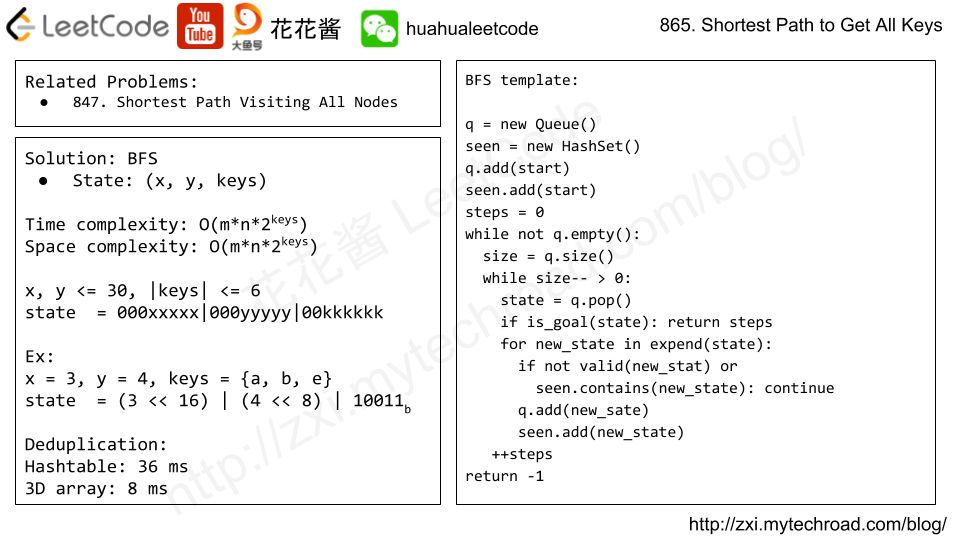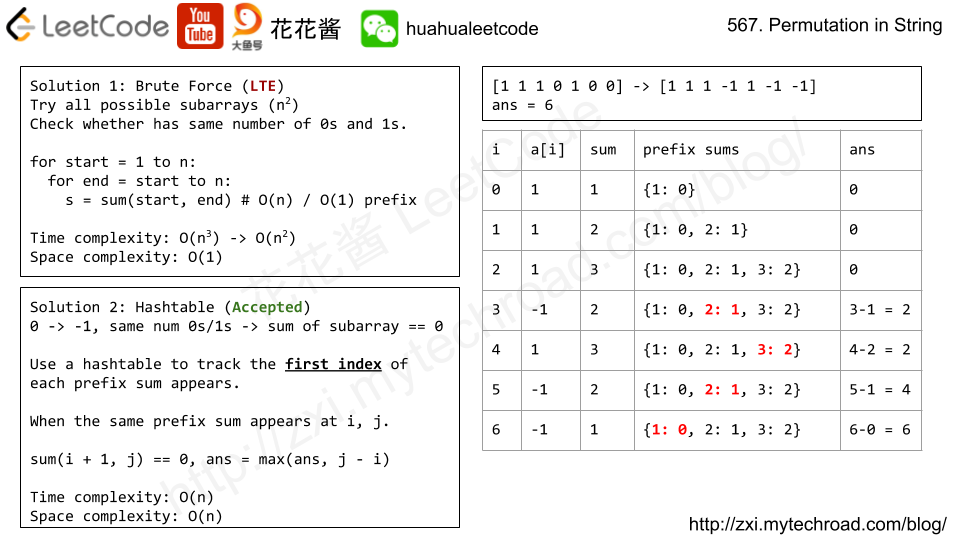# Problem

A sequence X_1, X_2, ..., X_n is fibonacci-like if:

• n >= 3
• X_i + X_{i+1} = X_{i+2} for all i + 2 <= n

Given a strictly increasing array A of positive integers forming a sequence, find the length of the longest fibonacci-like subsequence of A.  If one does not exist, return 0.

(Recall that a subsequence is derived from another sequence A by deleting any number of elements (including none) from A, without changing the order of the remaining elements.  For example, [3, 5, 8] is a subsequence of [3, 4, 5, 6, 7, 8].)

Example 1:

Input: [1,2,3,4,5,6,7,8]
Output: 5
Explanation:
The longest subsequence that is fibonacci-like: [1,2,3,5,8].


Example 2:

Input: [1,3,7,11,12,14,18]
Output: 3
Explanation:
The longest subsequence that is fibonacci-like:
[1,11,12], [3,11,14] or [7,11,18].


Note:

• 3 <= A.length <= 1000
• 1 <= A < A < ... < A[A.length - 1] <= 10^9
• (The time limit has been reduced by 50% for submissions in Java, C, and C++.)

# Solution 2: HashTable

Time complexity: O(n^3)

Space complexity: O(n)

# Problem

Starting with a positive integer N, we reorder the digits in any order (including the original order) such that the leading digit is not zero.

Return true if and only if we can do this in a way such that the resulting number is a power of 2.

Example 1:

Input: 1
Output: true


Example 2:

Input: 10
Output: false


Example 3:

Input: 16
Output: true


Example 4:

Input: 24
Output: false


Example 5:

Note:

1. 1 <= N <= 10^9

# Solution: HashTable

Compare the counter of digit string with that of all power of 2s.

e.g. 64 -> {4: 1, 6: 1} == 46 {4:1, 6: 1}

Time complexity: O(1)

Space complexity: O(1)

C++

# Problem

Given two arrays, write a function to compute their intersection.

Example:
Given nums1 = [1, 2, 2, 1]nums2 = [2, 2], return [2, 2].

Note:

• Each element in the result should appear as many times as it shows in both arrays.
• The result can be in any order.

• What if the given array is already sorted? How would you optimize your algorithm?
• What if nums1‘s size is small compared to nums2‘s size? Which algorithm is better?
• What if elements of nums2 are stored on disk, and the memory is limited such that you cannot load all elements into the memory at once?

# Solution1: Hashtable

Time complexity: O(m + n)

Space complexity: O(m)

# Problem

We are given a 2-dimensional grid"." is an empty cell, "#" is a wall, "@" is the starting point, ("a""b", …) are keys, and ("A""B", …) are locks.

We start at the starting point, and one move consists of walking one space in one of the 4 cardinal directions.  We cannot walk outside the grid, or walk into a wall.  If we walk over a key, we pick it up.  We can’t walk over a lock unless we have the corresponding key.

For some 1 <= K <= 6, there is exactly one lowercase and one uppercase letter of the first K letters of the English alphabet in the grid.  This means that there is exactly one key for each lock, and one lock for each key; and also that the letters used to represent the keys and locks were chosen in the same order as the English alphabet.

Return the lowest number of moves to acquire all keys.  If it’s impossible, return -1.

Example 1:

Input: ["@.a.#","###.#","b.A.B"]
Output: 8


Example 2:

Input: ["@..aA","..B#.","....b"]
Output: 6


Note:

1. 1 <= grid.length <= 30
2. 1 <= grid.length <= 30
3. grid[i][j] contains only '.''#''@''a'-'f' and 'A'-'F'
4. The number of keys is in [1, 6].  Each key has a different letter and opens exactly one lock.# Solution: BFS

Time complexity: O(m*n*64)

Space complexity: O(m*n*64)

# Problem

Given a binary array, find the maximum length of a contiguous subarray with equal number of 0 and 1.

Example 1:

Input: [0,1]
Output: 2
Explanation: [0, 1] is the longest contiguous subarray with equal number of 0 and 1.


Example 2:

Input: [0,1,0]
Output: 2
Explanation: [0, 1] (or [1, 0]) is a longest contiguous subarray with equal number of 0 and 1.


Note: The length of the given binary array will not exceed 50,000.# Solution: HashTable

Prefix sum + hashtable

Time complexity: O(n)

Space complexity: O(n)

V2: Using array instead of a hashtable

Time complexity: O(2*n + 1 + n)

Space complexity: O(2*n + 1)

Mission News Theme by Compete Themes.